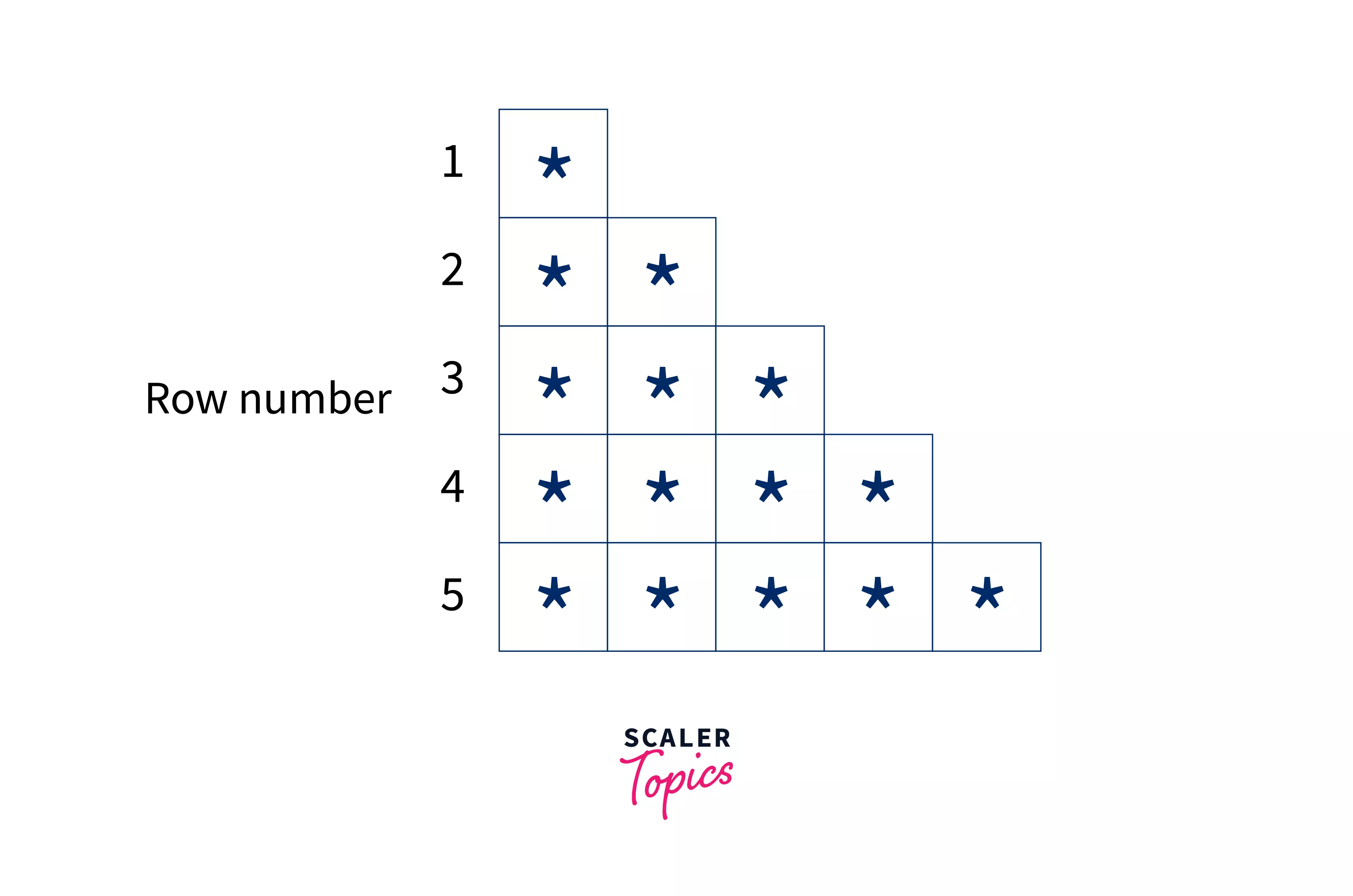# What is Nested For Loop in Python?

Challenge Inside! : Find out where you stand! Try quiz, solve problems & win rewards!
Learn via video coursePython Course for Beginners With Certification: Mastering the Essentials
By Rahul JanghuFree
4.90Enrolled: 1000Python Course for Beginners With Certification: Mastering the Essentials
Rahul JanghuFree
4.90
Enrolled: 1000

## What is Nested for loop in Python

Before moving to nested for loop, let's have a quick recap of for loop in python.
When we have to execute some statements multiple times, it is not efficient for us to write the same code repeatedly. So for loop helps us execute the statements multiple times here.

### Syntax of for loop in Python

The above for loop executes several times. If a = 3, the for loop will execute 3 times, if a = 10, the for loop will execute 10 times. Here x is the counter that is iterating on the range 0 to a-1.
The output of the above code for a = 3 is,

The range() function generates the sequence of numbers. Let's see how we can generate different types of a sequence using the range() function.

### range() in Python

If a single argument is provided to the range() function, the range function creates a sequence from 0 to a-1.

If two arguments are provided to the range() function, the range function creates a sequence from a to b-1.

If three arguments are provided to the range() function, the range function creates a sequence as a, a+c, a+2c, a+3c, ... a+nc till (a+nc)<b.

Now that we have a brief idea of a for loop let's move on to a nested for loop in Python.

Nested means one inside another, therefore nested for loop is a for loop inside another for loop. Or we can say we have a for loop inside the body of another for loop. Let's look over the syntax to see how nested loop is represented in python.

## Syntax of Using a Nested for loop in Python

The x loop or outer loop is executed several times. And for each execution of the x loop, the y loop is getting executed b number of times. Hence the statement of y loop is executing a*b number of times.
Let's understand the working of the nested for loop using an example.
The outer loop will iterate over the numbers in the range 0 to 4. And for each outer loop iteration, the inner loop will iterate from 5 to 9.

Output:

As you can see in the above output, the "Value of x: " statement is printed 5 times. While the "Value of y: " statement is printed 5*5 = 25 times.

If you observe the above output, you can notice that the above output is in form of a 2-D matrix, hence the nested for loops can be used to iterate over 2-D matrices.

## Examples

### Use a nested for loop to find the prime numbers from 2 to 100

First, let's see how we can use a nested loop to find prime numbers from 2 to 100. The outer loop should iterate over the numbers in the range [2, 100]. And the inner loop checks if the current number is a prime number or not. If the number is divisible by any number in the range 2 to number-1, it is not a prime number. If the current number is a prime number, we print the current number.

Output:

### Nested for loop to Print the Pattern

Consider the pattern for n=5 rows.

If we carefully observe, in each row, we are printing the * row number times, like if the row number is 1, we print * 1 time. If the row number is 2, we print * 2 times. If the row number is 3 we print * 3 times, and so on.Now, let's see how we can break this problem in the form of a nested loop. For n=5, there are 5 rows; therefore, we need a loop to iterate over the rows. Hence, the outer loop should iterate over the rows. In each row, * is printed row number times. Therefore the inner loop should iterate row number times and print * in each inner loop execution. Once the row is printed, a new line should be printed.
But note that the range(a) generates a sequence from 0 to a-1, but our row number starts from 1 to n; hence we will pass two arguments to the range function.

Output:

### Nested for loop Program to Print Multiplication Table

For a number n, we have to generate a multiplication table of size n*n. Let n=5; then we have to generate the multiplication table as,

Like the above problem, the outer loop is iterating over rows from row=1 to row=n. In each outer loop iteration, the inner loop runs n times from col=1 to col=n. In each execution of the inner loop, we print the product of the row number and column number. Once the row is printed, a new line should be printed.
But note that the row and column numbers also start from 1, instead of 0. Hence we will pass two arguments to the range function.

Output:

:::

## Conclusion

• A nested loop is a loop inside a loop. Therefore a nested for loop is a for loop inside another for loop.
• The inner for loop is executed for each execution of the outer for loop. If the outer for loop has range(a) and the inner for loop has range(b), then the inner for loop executes a*b times.
• Nested for loops can be used to solve problems such as prime numbers in a given range, pattern printing, multiplication table printing, etc.